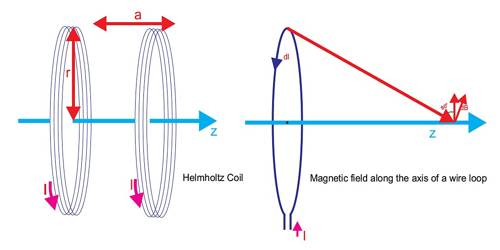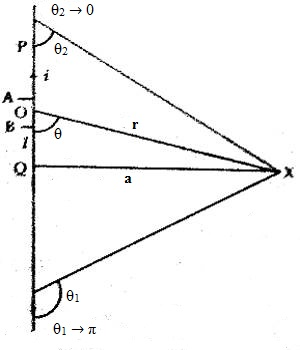Physics

# Applications of Biot-Savart’s LawBiot-Savart’s law is an equation that gives the magnetic field produced due to a current-carrying segment.

Applications of Biot-savart’s law

There are different applications of Biot-Savart’s law in electromagnetism. Two of them are discussed below:

(a) Magnetic induction at a point due to a long straight wire carrying current

(b) Magnetic induction at the center of a circular coil carrying current.

(a) Magnetic induction or magnetic field at a point due to a long straight wire carrying current: Suppose PQ is a long and straight wire and ‘i’ ampere current is flowing through it. Magnetic induction or magnetic field at point X at a distance r from the wire is to be determined.Let a very small section AB of that wire be taken. Let its length be dl. Suppose the distance from the center point O of that portion to the point X is r and angle ˂XOQ = θ.

So, the magnitude of the magnetic field obtained by solving the Biot-Savart Law for current element point X is,

B = μ0I / 2πa

Investigates: On which quantity the magmatic intensity prot1uced for a current-carrying wire or for magmatic poles depends.

Magnetic field intensity produced due to a current-carrying wire does not depend on the surrounding medium. But magnetic field intensity produced due to magnetic poles is dependent on the surrounding medium. The magnetic field produced along the length of the wire is zero.

(b) Magnetic induction or magnetic field at the center of the circular coil carrying current: Suppose magnetic field is produced around an infinitely small portion dl meter of a conductor due to the flow of ‘i’ ampere current through it. Let the distance of a point be r meter from the mid-point of this portion of the wire. If this distance makes an angle ‘α’ with the direction of flow of current, then according to the Biot-Savart’s law the magnetic induction or magnetic field at that point is,

dB = (μ0/4π) (idl/r2) sin α

Now, suppose ‘i’ ampere current is flowing through one turn of the circular wire radius of ‘r’ meter [Figure]. Magnetic induction at the center of the coil is to be determined due to the flow of current through the coil.According to the description and Biot-Savart’s law magnetic field produced at the center of the wire due to flow of current through the small portion of length ‘dl’ of the conductor is,

dB = (μ0idl sin α) / (4π r2) = [μ0 (idl sin 900) / (4π r2)] = μ0 (idl/4π r2) … … … (1)

Here sin α = sin 900, since the tangent at the mid-point of that portion and the radius drawn at that point are mutually perpendicular. Of course, this magnetic field will act normal to the plane that contains the circular conductor.

Resultant magnetic induction due to the whole circular wire will be equal to the sum of the magnetic induction produced due to such small portions of the wire. This is due to the fact that all the magnetic inductions will be in the same direction. So, the total magnetic induction due to the current flowing in the whole wire is obtained by integrating the equation (1) within limits from, l = 0 to l = bπr

So, B = (μ0/4π) (i/r2) 2πr0 dl = μ0i/2r

Instead of one turn if ‘i’ ampere torrent flow, through ‘n’ turns of the coil of radius r, then the magnetic induction or magnetic field will be increased ‘n’ times. So in that case magnetic induction,

B = [μ0ni/2r] Wb.m-2.

Some other applications of Biot Savart Law include the following –

• This law can be used for calculating magnetic reactions even on the level of molecular or atomic.
• It can be used in the theory of aerodynamic for determining the velocity encouraged with vortex lines.